# Texas Go Math Grade 2 Lesson 3.3 Answer Key Build Numbers in Different Ways

Refer to our Texas Go Math Grade 2 Answer Key Pdf to score good marks in the exams. Test yourself by practicing the problems from Texas Go Math Grade 2 Lesson 3.3 Answer Key Build Numbers in Different Ways.

## Texas Go Math Grade 2 Lesson 3.3 Answer Key Build Numbers in Different Ways

Explore

Draw quick pictures to solve. Write how many 1 hundreds, tens, and ones.
__________ hundred _________ tens ____________ ones
__________ hundred _________ tens ____________ ones

FOR THE TEACHER • Read this problem to children. Mrs. Walters wants to buy exactly 275 oranges for a community project. She can buy oranges in boxes of 100, bags of 10, and as single oranges. What are two different ways she can buy the oranges?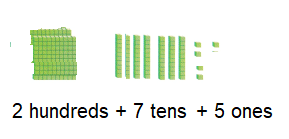Explanation:
2 hundred 7 tens 5 ones
oranges in 2 boxes of 100, 7 bags of 10, and as 5 single oranges
200 + 70 + 5 = 275
Math Talk
Mathematical Processes

Describe another way to show the number.
200 + 70 + 5 or 275
Explanation:
2 hundreds + 7 tens + 5 ones = 275
20 tens + 7 tens + 5 ones 275

Model and Draw

Here are two different ways that show the same number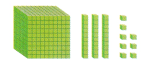__________thousands + __________ hundreds + __________tens + __________ones__________thousands + __________ hundreds + __________tens + __________ones
The number is __________.
Explanation: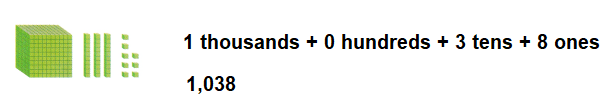Share and Show

Use blocks to show the same number a different way. Draw a quick picture of your model. Write the numbers.

Question 1.__________thousands + __________ hundreds + __________tens + __________ones
__________thousands + __________ hundreds + __________tens + __________ones
The number is __________thousands.
Explanation:
10 hundreds is 1 thousand
1 thousands + 0 hundreds + 2 tens + 5 ones
1 thousands + 2 tens + 5 ones
So, the number is 1,025.

Question 2.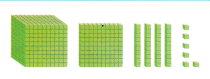__________thousands + __________ hundreds + __________tens + __________ones
__________thousands + __________ hundreds + __________tens + __________ones
The number is __________.
Explanation:
The above blocks in the question represents
1 thousands + 1 hundreds + 4 tens + 6 ones
As 1 thousand is 10 hundreds and 1 hundred is 10 tens
So, the number is 1,146.

Problem Solving

Use blocks to show the same number in two other ways. Draw quick pictures. Write the number.

Question 3.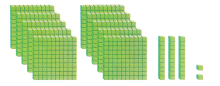__________thousands + __________ hundreds + __________tens + __________ones
__________thousands + __________ hundreds + __________tens + __________ones
__________thousands + __________ hundreds + __________tens + __________ones
__________thousands + __________ hundreds + __________tens + __________ones
The number is __________.
Explanation:
The above blocks in the question represents
1 thousands  + 3 tens + 2 ones
10 hundreds + 3 tens + 2 ones ( 1 thousand is 10 hundreds)
10 hundreds are arranged in 2two 5 hundreds
5 hundreds + 5 hundreds + 3 tens + 2 once
So, the number is 1032.

Solve. Write or draw to explain.

Question 4.
H.O.T. I have 10 hundreds blocks, 10 tens blocks, and 10 ones blocks. I use all my blocks to make a number. What is my number?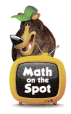Explanation:
I have 10 hundreds blocks, 10 tens blocks, and 10 ones blocks.
10 hundreds +10 tens + 10 ones
Use all the blocks to make a number
1,000 + 100 + 10 = 1,110

Question 5.
H.O.T. Multi-Step I have 9 hundreds blocks, 3 tens blocks, and 4 ones blocks. I get 5 more tens blocks and 2 more hundreds blocks. I use all my blocks to make a number. What is my number?
Explanation:
I have 9 hundreds blocks, 3 tens blocks, and 4 ones blocks.
9 hundreds + 3 tens + 4 ones = 934
I get 5 more tens blocks and 2 more hundreds blocks.
2 hundreds + 5 tens = 250
total blocks arranged together
934 + 250 =1,184

Question 6.
Representations Wendy modeled a number with 10 hundreds blocks and 11 tens blocks. What number did she model?
(A) 1,110
(B) 1,011
(C) 111
Option (A)
Explanation:
10 hundreds is 1 thousand,
10 tens is 1 hundred.
10 hundreds + 11 tens =
= 1000 +110
= 1,110

Question 7.
Use blocks to show the same number in a different way. Draw o quick picture of your model. Write the number.__________thousands + __________ hundreds + __________tens + __________ones
__________thousands + __________ hundreds + __________tens + __________ones
The number is __________.
Explanation:
Ten hundreds is 1 thousand, 10 tens is 1 hundred
1 thousands + 1 hundreds + 1 tens + 15 ones
1 thousands + 1 hundreds + 2 tens + 5 ones
So, the number is 1,125

Question 8.
TEXAS Test Prep Which shows the same number?(A) 1,063
(B) 1,163
(C) 1,073
Explanation:
The given blocks represents,
11 hundreds and 6 tens and 3 ones
= 1,163

TAKE HOME ACTIVITY • Have your child explain how he or she solved one of the exercises in this lesson.

### Texas Go Math Grade 2 Lesson 3.3 Homework and Practice Answer Key

Use blocks to show the same number a different way. Draw a quick picture of your model. Write the numbers.

Question 1.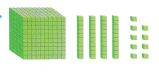__________thousands + __________ hundreds + __________tens + __________ones
__________thousands + __________ hundreds + __________tens + __________ones
The number is __________.
Explanation:
1 thousands + 3 tens + 9 ones
10 hundreds + 39 ones
So, the number is 1,039.

Question 2.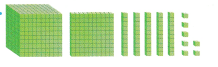__________thousands + __________ hundreds + __________tens + __________ones
__________thousands + __________ hundreds + __________tens + __________ones
The number is __________.
Explanation:
1 thousands + 1 hundred + 5 tens + 7 ones
11 hundreds + 57 ones
So, the number is 1,157.

Problem Solving

Solve.

Question 3.
Multi-Step I have 7 hundreds blocks, 4 tens blocks, and 5 ones blocks. I get 2 more tens blocks, and 3 more hundreds blocks. I use all my blocks to make a number. What is my number?
Explanation:
7 hundreds + 4 tens + 5 ones = 745
3 hundreds + 2 tens = 320
total
745 + 320 =1,065

Lesson Check

Question 4.
Which shows the same number?(A) 1,032
(B) 132
(C) 1,123
Option (A)
Explanation:
The given picture has 2 sets of 5 hundred blocks
500 + 500 + 30 + 2
10 hundred blocks is 1 thousand
= 1000 + 30 + 2 = 1,032

Question 5.
Ken is modeling the number 1,172. He does not use any thousands. How many hundreds will he use?
(A) 11
(B) 7
(C) 20
Option (A)
Explanation:
1,172 is a four digit number 1000 + 100 = 1100
it means 11 hundreds are used by Ken,
as ten hundreds is equal to 1 thousand.

Question 6.
Lena uses red blocks to show 0 thousands,10 hundreds, 0 tens, 36 ones.
She uses blue blocks to show 1 thousand, 1 hundred, 3 tens, 6 ones.
She uses yellow blocks to show 1 thousand, 13 tens, 6 ones.
Which color blocks show the same number?
(A) red blocks and blue blocks
(B) blue blocks and yellow blocks
(C) red blocks and yellow blocks